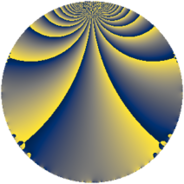# Properties

 Label 91.2.bbLevel $91$ Weight $2$ Character orbit 91.bb Rep. character $\chi_{91}(5,\cdot)$ Character field $\Q(\zeta_{12})$ Dimension $32$ Newform subspaces $1$ Sturm bound $18$ Trace bound $0$

# Related objects

## Defining parameters

 Level: $$N$$ $$=$$ $$91 = 7 \cdot 13$$ Weight: $$k$$ $$=$$ $$2$$ Character orbit: $$[\chi]$$ $$=$$ 91.bb (of order $$12$$ and degree $$4$$) Character conductor: $$\operatorname{cond}(\chi)$$ $$=$$ $$91$$ Character field: $$\Q(\zeta_{12})$$ Newform subspaces: $$1$$ Sturm bound: $$18$$ Trace bound: $$0$$

## Dimensions

The following table gives the dimensions of various subspaces of $$M_{2}(91, [\chi])$$.

Total New Old
Modular forms 48 48 0
Cusp forms 32 32 0
Eisenstein series 16 16 0

## Trace form

 $$32 q - 2 q^{2} - 12 q^{3} - 6 q^{5} - 6 q^{7} - 16 q^{8} + 8 q^{9} + O(q^{10})$$ $$32 q - 2 q^{2} - 12 q^{3} - 6 q^{5} - 6 q^{7} - 16 q^{8} + 8 q^{9} - 10 q^{11} + 28 q^{14} - 44 q^{15} + 12 q^{16} - 4 q^{18} + 12 q^{19} - 26 q^{21} - 8 q^{22} - 12 q^{24} + 24 q^{26} - 6 q^{28} + 16 q^{29} + 24 q^{31} + 4 q^{32} + 48 q^{33} + 28 q^{35} - 8 q^{37} - 6 q^{39} - 132 q^{40} - 16 q^{42} - 42 q^{44} - 24 q^{45} + 12 q^{46} + 30 q^{47} + 88 q^{50} + 36 q^{52} - 12 q^{53} + 78 q^{54} + 40 q^{57} + 26 q^{58} - 54 q^{59} + 16 q^{60} - 48 q^{61} + 24 q^{63} - 8 q^{65} + 12 q^{66} + 16 q^{67} - 48 q^{68} + 50 q^{70} - 36 q^{71} + 22 q^{72} + 66 q^{73} + 12 q^{74} - 176 q^{78} - 32 q^{79} + 138 q^{80} + 16 q^{81} - 58 q^{84} - 84 q^{85} + 42 q^{86} - 24 q^{87} - 60 q^{89} + 48 q^{92} + 6 q^{93} - 72 q^{94} - 42 q^{96} - 86 q^{98} - 24 q^{99} + O(q^{100})$$

## Decomposition of $$S_{2}^{\mathrm{new}}(91, [\chi])$$ into newform subspaces

Label Dim $A$ Field CM Traces $q$-expansion
$a_{2}$ $a_{3}$ $a_{5}$ $a_{7}$
91.2.bb.a $32$ $0.727$ None $$-2$$ $$-12$$ $$-6$$ $$-6$$